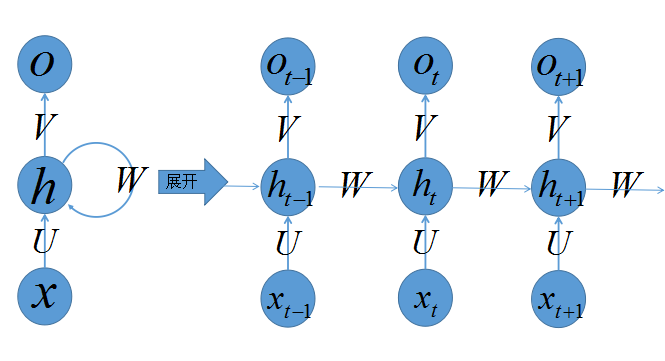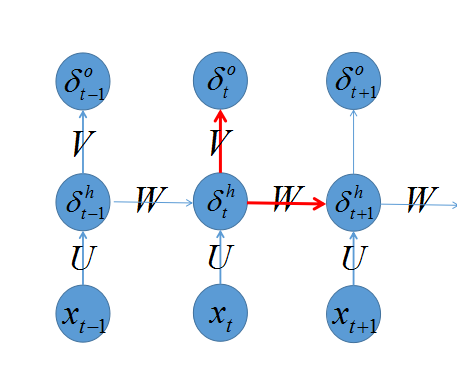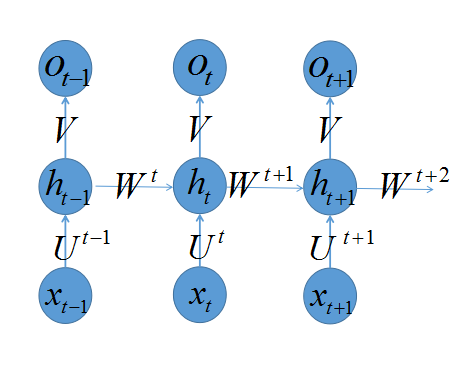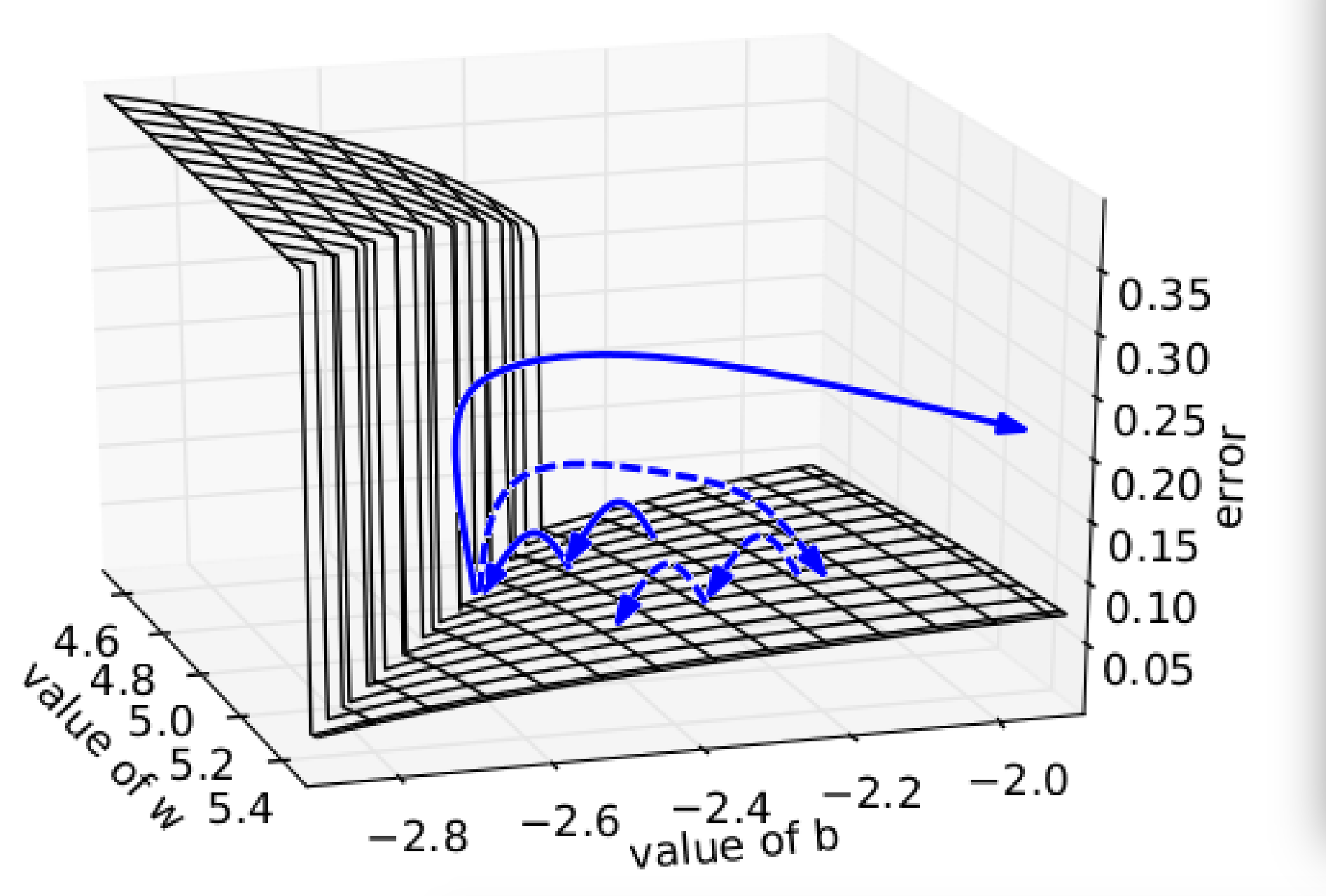RNN的基本结构• 网络某一时刻的输入xt$x_t$，和之前介绍的多层感知器的输入一样，xt$x_t$是一个n$n$维向量，不同的是递归网络的输入将是一整个序列，也就是x=[x1,...,xt1,xt,xt+1,...xT]$x=[x_1,...,x_{t-1},x_{t},x_{t+1},...x_T]$，对于语言模型，每一个xt$x_t$将代表一个词向量，一整个序列就代表一句话。
• ht$h_t$代表时刻t$t$的隐藏状态
• ot$o_t$代表时刻t$t$的输出
• 输入层到隐藏层直接的权重由U$U$表示，它将我们的原始输入进行抽象作为隐藏层的输入
• 隐藏层到隐藏层的权重W$W$，它是网络的记忆控制者，负责调度记忆。
• 隐藏层到输出层的权重V$V$，从隐藏层学习到的表示将通过它再一次抽象，并作为最终输出。

RNN的Forward阶段

s1=Ux1+Wh0h1=f(s1)o1=g(Vh1)

s2=Ux2+Wh1h2=f(s2)o2=g(Vh2)
，以此类推
st=Uxt+Wht1ht=f(Uxt+Wht1)ot=g(Vht)

h=f(+)

RNN的Backward阶段

U=EU=tetUV=EV=tetVW=EW=tetWδht=(VTδot+WTδht+1).f(st)

W=tδh0,th0,t1,...,δh0,thi,t1,...,δh0,thm,t1...δhj,th0,t1,...,δhj,thi,t1,...,δhj,thm,t1...δhn,th0,t1,...,δhn,thi,t1,...,δhn,thm,t1=tδht×ht1U=tδh0,tx0,t,...,δh0,txi,t,...,δh0,txm,t...δhj,tx0,t,...,δhj,txi,t,...,δhj,txm,t...δhn,tx0,t,...,δhn,txi,t,...,δhn,txm,t=tδht×xt

RNN的训练困难et+1Wt+1=et+1ht+1ht+1Wt+1
et+1Wt=et+1ht+1ht+1hthtWt
et+1Wt1=et+1ht+1ht+1hththt1ht1Wt1
......

etW=1ktethtk<ithihi1+hkW

f(h(x))x=f(h(x))

||k<ithihi1||ηtk参考引用